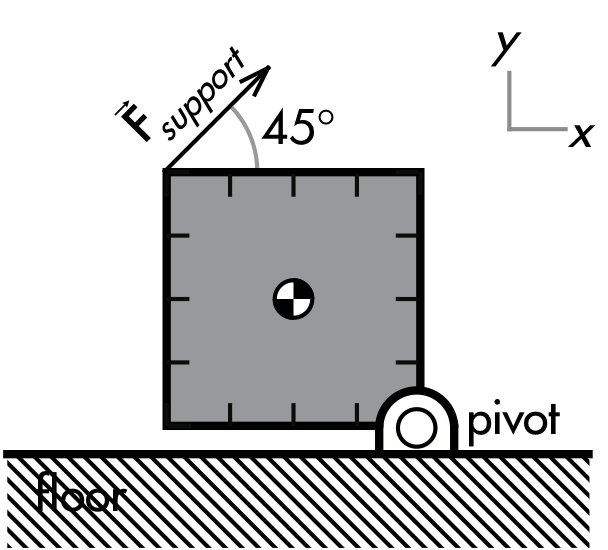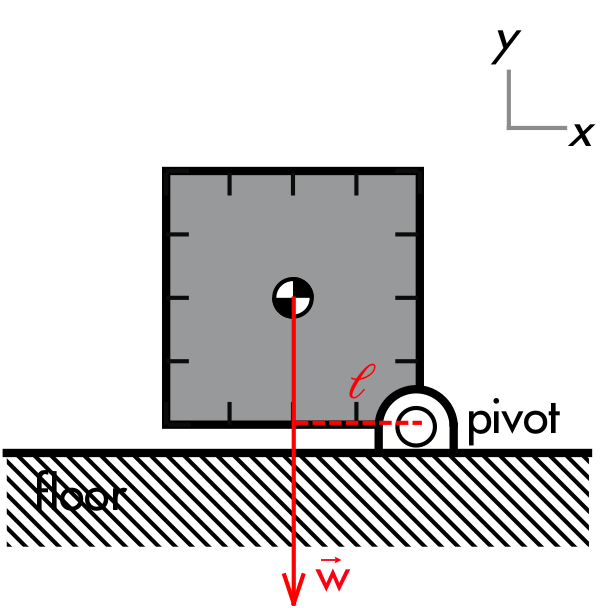## 20191104

### Physics quiz question: weight torque on supported square

Physics 205A Quiz 5, fall semester 2019
Cuesta College, San Luis Obispo, CAA uniform 0.40 m × 0.40 m square with  a mass of 0.50 kg is pivoted at one corner. It is supported by a 45° diagonal force at the opposite corner such that the bottom edge is parallel to the ground. Calculate all torques with respect to the corner pivot. The magnitude of the torque exerted by the weight force is:
(A) 0.98 N·m.
(B) 1.4 N·m.
(C) 2.0 N·m.
(D) 2.8 N·m.

Correct answer (highlight to unhide): (A)The weight force w of Earth acting on the beam acts at the center of gravity, directly downwards with a magnitude m·g. The perpendicular lever arm ℓ for the weight force on the beam must extend from the pivot point to perpendicularly intercept the weight force line of action (which lies along the weight force vector), such that this will be a horizontal line that is half the side of the square:

ℓ = 0.20 m.

The magnitude of the (counterclockwise) torque exerted by the weight force on the beam is then:

τ = w·ℓ,

τ = (m·g)·(0.20 m),

τ = ((0.50 kg)·(9.80 m/s2))·(0.20 m),

τ = 0.98 N·m.

(Response (B) is m·g·(0.20 m)/sin(45°); response (C) is m·g·(0.40 m); and response (D) is m·g·(0.40 m)/sin(45°).)

Sections 70854, 70855
Exam code: quiz05Gu1L
(A) : 26 students
(B) : 16 students
(C) : 6 students
(D) : 4 students

Success level: 50%
Discrimination index (Aubrecht & Aubrecht, 1983): 0.65